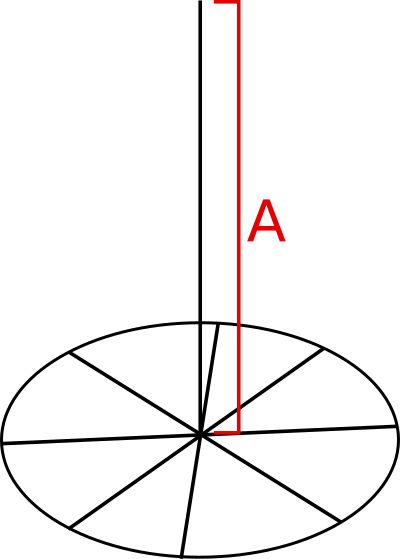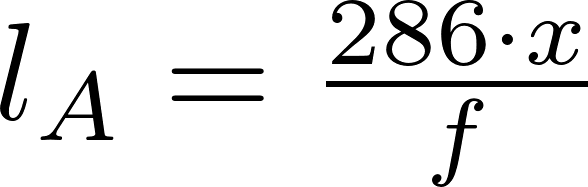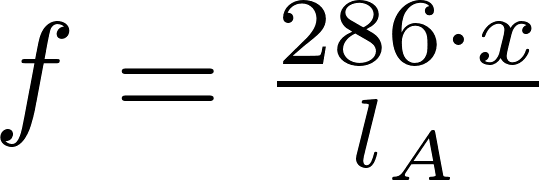# Vertical antenna

The vertical antenna is a very simple antenna consisting of a vertical rod.

### FormulasSchematicl is the symbol for length and is measured in meter (m).
f is the frequency in megahertz (MHz).
x is the wavelength fraction (e.g. 1/4 λ = 0.25).

### Calculator

Enter two values to calculate the third.